Next: Observables and other characteristic Up: Observables and single particle Previous: Hartree-Fock equivalent energies, radii,

## Canonical basis

The canonical states are obtained by diagonalizing the density matrix (see e.g. Refs. [10,18] for the interpretation of the canonical basis)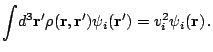(50)

As discussed in Refs. [5,18], all canonical states have localized wave functions and form a basis. The energies of the canonical states are defined as the diagonal matrix elements of the Hartree-Fock field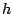in the canonical basis,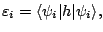(51)

and the pairing gaps associated with these states are the diagonal matrix elements of the pairing field,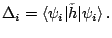(52)

Finally, the canonical quasiparticle energy can be defined using the same kind of formula as (48),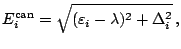(53)

while the occupation probabilities of canonical states are given by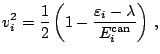(54)

These characteristics of the canonical states can be found in the output file hfb_n_p.spe, see Sec. 7.Next: Observables and other characteristic Up: Observables and single particle Previous: Hartree-Fock equivalent energies, radii,
Jacek Dobaczewski 2005-01-23